# Modular Arithmetic

We have some articles in this blog about integers of typical programming languages and how they work. Time to introduce the underlying mathematical concepts, that have been covered implicitly until now, since they are also interesting in many other aspects. And besides, this is a very beautiful area of mathematics.

Mathematics that we learn in school is mostly inspired by what is needed for physics. This was quite a good choice 100 years ago, because it gave some motivation to why we do certain things and it was the area, where math was applied. Of course also chemistry and engineering, but these are somewhat similar aspects of mathematics as we use in physics. Now physics and chemistry make use of quite interesting areas of mathematics like group theory or non Euclidean geometry, but these are kind of advanced areas beyond what we typically learn in school. at least in the countries where I went to school. So it is about real numbers, some trigonometry, real analysis (calculus) and maybe complex numbers.

Since more than 50 years mathematics is heavily used in informatics as well, if we abstract informatics away from computers, even longer, because for example algorithms and cryptography have been used for several thousand years already, but that was a small niche and became main stream by the existence of computers. And for informatics and computer science we need different areas of mathematics. Analysis is not the so important, though not irrelevant. One area is information theory, which is based on probability theory and statistics. Numerical calculations have to a great extent remained a domain of mathematics itself, so this connection may be strong, but it is applied mathematicians using computers and using knowledge from IT to program them better, not the other way round. Still numerical analysis is somewhat important, but not really what most of us need very often.

The areas of mathematics that are really interesting for informatics are discrete mathematics, algebra and number theory. There is enough material about this on the web, but for now we will deal with modular arithmetic, which is kind of in the intersection of discrete mathematics, algebra and number theory.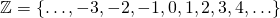Now we take any positive integral number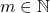with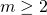.
We say that two integral numbers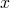and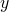are congruent modulo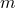: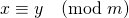if and only if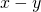can be divided by. We might also say that there is a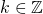such that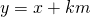.
Now we can make interesting observations:
We assume, that we have pairs of numbers such that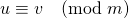andThen we can observe that also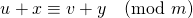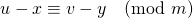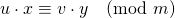This can be proven easily.
We assume as above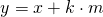and similarly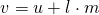Then we have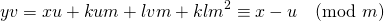We call a set of all numbers of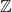that are congruent to each other a remainder class and write this as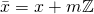There are exactlyremainder classes moduloand usually we use a representation system of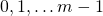or for evenwe often use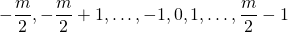or for oddwe often use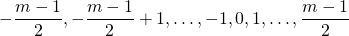We observe these representation systems when we do division with remainder, written as % in many programming languages, but it is necessary to do some quick research on which representation system % uses and which one we want to use and possibly adjust the result. The corresponding division may not be /, but we can obtain it by subtracting our remainder from the dividend and dividing that, which should be an exact division.

Now we need to define a ring. A ring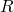is a set with operations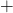andsuch that the following rules apply:

1. For any members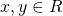we also have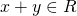,and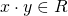. This is usually not mentioned, because it is part of how we define these operations in the first place in most mathematical texts.
2. Addition is communicative: For any memberswe have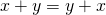.
3. Addition has a neutral element 0: For any memberwe have.
4. Addition has inverse elements: For any memberwe have a membersuch that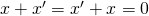. Usually we write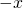for this inverse element ofand we writeinstead of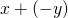.
5. Addition is associative: For any members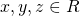we have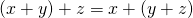. We can omit the parentheses here and write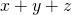instead.
6. Multiplication has a neutral element 1: For any memberwe have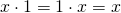.
7. Multiplication is associative: For any memberswe have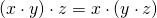. We can omit the parentheses here and writeor even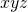instead.
8. Multiplication in conjunction with addition is distributive: For any memberswe have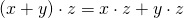and.

If the multiplication is also communicative, we call it a commutative ring. If there is a multiplicative inverse for any element other than 0, we call it a skew field. And if both conditions hold, we call it a field.

Now we can see thatis actually a communicative ring.

And these remainder classes moduloalso form a ring. We call it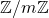or sometimes also, but I do not use the second form, because it is ambiguous with something else (p-adic numbers). If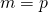is a prime number, then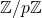is actually a field and in this case we may writeinstead of. Or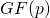in some literature, if you prefer that. Why is it a field?

Now we have an extension of the Euclid algorithm to calculate the gcd of two numbers. This also yields numbers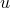and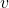such that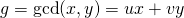. So these numbers exist. For a prime number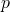and a remainder class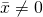we know thatis not a multiple ofand sinceis prime we know that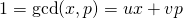. This yields a multiplicative inverse for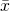because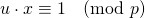.

Now we often seeas a power of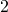and the modular arithmetic, at least +, -, *, is what is sold to us as integer arithmetic of Java, C or C#.

On the other hand it can be interesting and useful to use modular arithmetic for other values of. Interesting are mostly prime numbers, which can be relatively small like,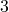or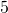, but also really big. For non-primes we have null-factors, that is numberssuch that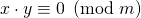. This breaks some fundamental mathematical assumption for integers and fields, but is perfectly correct for this modular ring.

In our daily life modular arithmetic is actually quite common. We have the week days with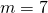, the hours of the clock withor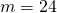, the minutes and seconds of the clock with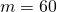and quite a bit of, which we do not really see as modular arithmetic, but maybe as boolean arithmetic withbeing the „exclusive or“,being the „and“ etc.